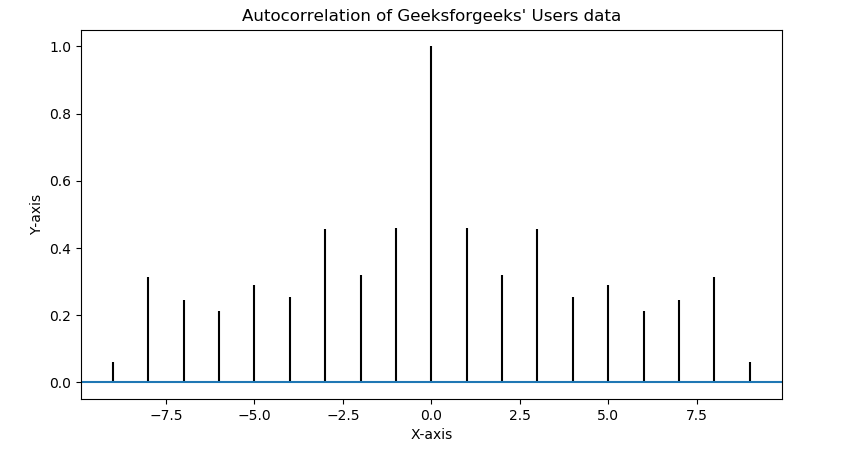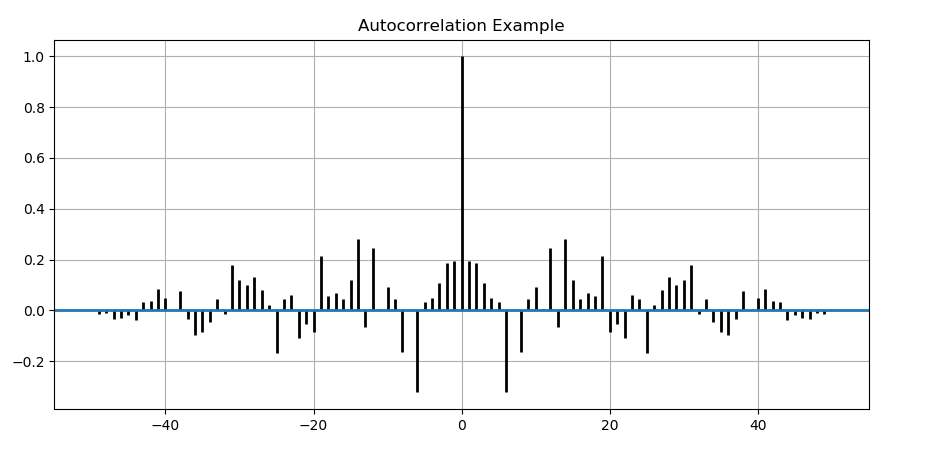# Matplotlib.pyplot.acorr() in Python

Matplotlib is a library in Python and it is numerical – mathematical extension for NumPy library. Pyplot is a state-based interface to a Matplotlib module which provides a MATLAB-like interface.

## matplotlib.pyplot.acorr() Function

The acorr() function in pyplot module of matplotlib library is used to plot the autocorrelation of x (array-like).

Syntax: matplotlib.pyplot.acorr(x, *, data=None, **kwargs)

Parameters: This method accept the following parameters that are described below:

• x: This parameter is a sequence of scalar.
• detrend: This parameter is an optional parameter. Its default value is mlab.detrend_none
• normed: This parameter is also an optional parameter and contains the bool value. Its default value is True
• usevlines: This parameter is also an optional parameter and contains the bool value. Its default value is True
• maxlags: This parameter is also an optional parameter and contains the integer value. Its default value is 10
• linestyle: This parameter is also an optional parameter and used for plotting the data points, only when usevlines is False.
• marker: This parameter is also an optional parameter and contains the string. Its default value is ‘o’

Returns: This method returns the following:

• lags:This method returns the lag vector
• c:This method returns the auto correlation vector.
• line : Added LineCollection if usevlines is True, otherwise add Line2D.
• b: This method returns the horizontal line at 0 if usevlines is True, otherwise None.

The resultant is (lags, c, line, b).

Below examples illustrate the matplotlib.pyplot.acorr() function in matplotlib.pyplot:

Example #1:

 `# Implementation of matplotlib.pyplot.acorr() ` `# function ` ` `  `import` `matplotlib.pyplot as plt ` `import` `numpy as np ` ` `  `# Time series data ` `geeks ``=` `np.array([``24.40``, ``110.25``, ``20.05``, ``22.00``, ` `                  ``61.90``, ``7.80``, ``15.00``, ``22.80``,  ` `                  ``34.90``, ``57.30``]) ` ` `  `# Plot autocorrelation ` `plt.acorr(geeks, maxlags ``=` `9``) ` ` `  `# Add labels to autocorrelation plot ` `plt.title(``"Autocorrelation of Geeksforgeeks' Users data"``) ` `plt.xlabel(``'X-axis'``) ` `plt.ylabel(``'Y-axis'``) ` ` `  `# Display the autocorrelation plot ` `plt.show() `

Output:Example #2:

 `# Implementation of matplotlib.pyplot.acorr()  ` `# function ` ` `  `import` `matplotlib.pyplot as plt ` `import` `numpy as np ` ` `  ` `  `# Fixing random state for reproducibility ` `np.random.seed(``10``*``*``7``) ` ` `  `geeks ``=` `np.random.randn(``51` `) ` ` `  `plt.title(``"Autocorrelation Example"``) ` `plt.acorr(geeks, usevlines ``=` `True``,  ` `          ``normed ``=` `True``, maxlags ``=` `50``,  ` `          ``lw ``=` `2``) ` ` `  `plt.grid(``True``) ` `plt.show() `

Output:Attention geek! Strengthen your foundations with the Python Programming Foundation Course and learn the basics.

To begin with, your interview preparations Enhance your Data Structures concepts with the Python DS Course.

My Personal Notes arrow_drop_upSmall things always make you to think big

If you like GeeksforGeeks and would like to contribute, you can also write an article using contribute.geeksforgeeks.org or mail your article to contribute@geeksforgeeks.org. See your article appearing on the GeeksforGeeks main page and help other Geeks.

Please Improve this article if you find anything incorrect by clicking on the "Improve Article" button below.

Article Tags :

Be the First to upvote.

Please write to us at contribute@geeksforgeeks.org to report any issue with the above content.